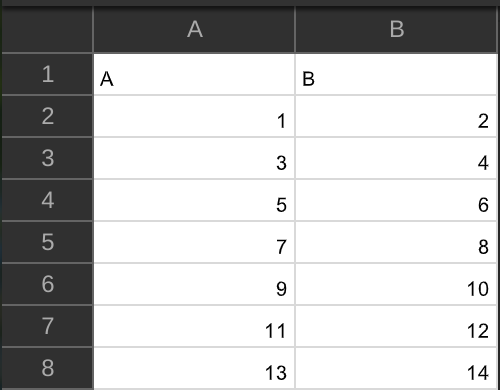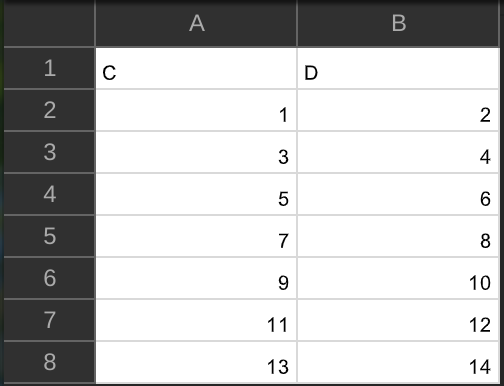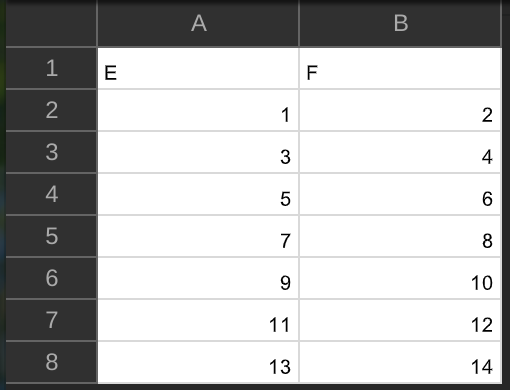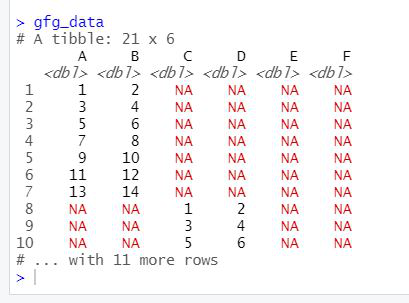# Import and Merge Multiple CSV Files in R

• Last Updated : 17 Jun, 2021

In this article, we will be looking at the approach to merge multiple CSV files in the R programming language.

### Modules Used

• dplyr: This is a structure of data manipulation that provides a uniform set of verbs, helping to resolve the most frequent data manipulation hurdles.
• plyr: plyr is an R package that makes it simple to split data apart, do stuff to it, and mash it back together.
• readr: This provides a fast and friendly way to read rectangular data (like ‘csv’, ‘tsv’, and ‘fwf’).

### Functions Used

• list.files() function: This function produces a character vector of the names of files or directories in the named directory.

Syntax: list.files(path = “.”, pattern = NULL, all.files = FALSE,full.names = FALSE, recursive = FALSE, ignore.case = FALSE, include.dirs = FALSE, no.. = FALSE)

• lapply() function: This function returns a list of the same length as X, each element of which is the result of applying FUN to the corresponding element of X.

Syntax: lapply(X, FUN, …)

• bind_rows() function: This function is an efficient implementation of the common pattern of do.call(rbind, dfs) or do.call(cbind, dfs) for binding many data frames into one.

Syntax:

bind_rows(…, .id = NULL)

Parameter:

…: Data frames to combine.

.id: Data frame identifier.

To merge multiple CSV files, the user needs to install and import dplyr,plyr, and readr packages in the R console to call the functions which are list.files(), lapply(), and bind_rows() from these packages and pass the required parameters to these functions to merge the given multiple CSV files to a single data frame in the R programming language.

Data in Use:Example:

## R

 `library``(``"dplyr"``)                                                ``library``(``"plyr"``)                                               ``library``(``"readr"``)  `` ` `gfg_data <- ``list.files``(path = ``"C:/Users/Geetansh Sahni/Documents/R/Data"``, ``                      ``pattern = ``"*.csv"``, full.names = ``TRUE``) %>%`` ``lapply``(read_csv) %>%                                          `` ``bind_rows                                                      `` ` `gfg_data`

Output:My Personal Notes arrow_drop_up